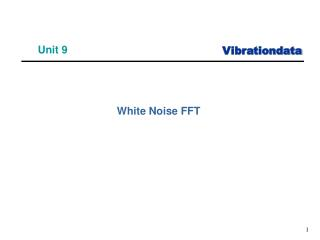Download PresentationUnit 9

Unit 9 - PowerPoint PPT Presentation

Unit 9. White Noise FFT. Fourier Transform, Sine Function. Review. A Fourier transform will give the exact magnitude and frequency for a steady-state sine function provided that no leakage error occurs The sine function must have an integer number of cycles to prevent leakageI am the owner, or an agent authorized to act on behalf of the owner, of the copyrighted work described.
Download PresentationUnit 9

Download Policy: Content on the Website is provided to you AS IS for your information and personal use and may not be sold / licensed / shared on other websites without getting consent from its author.While downloading, if for some reason you are not able to download a presentation, the publisher may have deleted the file from their server.

- - - - - - - - - - - - - - - - - - - - - - - - - - E N D - - - - - - - - - - - - - - - - - - - - - - - - - -
Presentation Transcript
1. Unit 9 • White Noise FFT

2. Fourier Transform, Sine Function Review . . . • A Fourier transform will give the exact magnitude and frequency for a steady-state sine function provided that no leakage error occurs • The sine function must have an integer number of cycles to prevent leakage • The same is true for an FFT if the time history has 2N points where N is an integer

3. White Noise • But how useful is the FFT for broadband random vibration such as white noise?

4. White Noise Generate white noise with the following parameters: • SR = 6553.6 samples/sec • 10 second duration • Std dev = 5 • 65536 samples • No band limit filter Then extract the 0 to 1.25 second segment from the 10-second time history

5. White Noise Each time history has std dev = 5.0

6. White Noise Top FFT: 1.25 second duration f=0.8 Hz Mean = 0.0971 Bottom FFT: 10 second duration f=0.1 Hz Mean = 0.0347 Difference in mean = 8

7. White Noise Comparison • Ideally, the "white noise" would have a constant Fourier transform magnitude with respect to frequency • The fact that there is some variation within each transform is unimportant for this example • The pertinent point is that the mean magnitude changes by 8 , comparing the two transforms • The reason for the decrease is that the transform in the 1.25 second figure has 4096 spectral lines compared to the 32768 spectral lines in the 10 secondfigure (up to Nyquist Frequency) • Thus, the "energy" is divided into a greater number of spectral lines in the 10 secondtransform

8. Fourier Magnitude, 1.25 second Record Recall time history synthesis , std dev = 5 (GRMS = std dev, for zero mean) First twelve row of Excel spreadsheet are shown. GRMS = G peak / sqrt(2) Use fill down to cover all 4096 rows.

9. Parseval’s Theorem x(t) is the time history X(f) is the Fourier transform The RMS value can either be calculated from the time history or the Fourier transform. The results is the same regardless.

10. Conclusion • The FFT magnitude is a poor tool for characterizing white noise magnitude! • Need a better tool for random vibration • That tool will be the Power Spectral Density (PSD) • PSD can be calculate from an FFT, but it expresses the energy as a density • PSD magnitude is mostly insensitive to duration except that a greater number of statistical-degrees-of-freedom are accumulated by taking a longer duration

11. Exercise • Perform the example in the main text yourself • Use the Vibrationdata GUI package & Excel • Note that the package has a function : • Signal Edit Utilities > Extract Segment